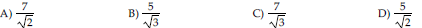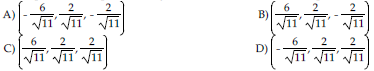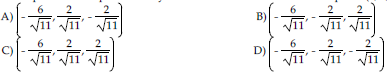/
/
/
271) A rectangular box with square base and no top
Not my Question
Flag Content

# Question : 271) A rectangular box with square base and no top : 1757208

271) A rectangular box with square base and no top is to have a volume of 32 ft3. What is the least amount of material required?

A) 42 ft2 B) 48 ft2 C) 40 ft2 D) 36 ft2

272) A rectangle with sides parallel to the axes is inscribed in the region bounded by the axes and the line x + 2y = 2. Find the maximum area of this rectangle.

A) 1/4 B) 2/3 C) 1/2 D) 1/3

273) Find the point on the plane x + 2y - z = 12 that is nearest the origin.

A) (4, 4, 0) B) (2, 4, 0) C) (-2, 8, 2) D) (2, 4, -2)

274) Find the distance from the point (1, -1, 2) to the plane x + y - z = 3.275) Find the point on the sphere x2 + y2 + z2 = 4 that is closest to the point (3, 1, -1).276) Find the point on the sphere x2 + y2 + z2 = 4 that is farthest from the point (3, 1, -1).277) A rectangular box is to be inscribed inside the ellipsoid 2x2 + y2 + 4z2 = 12. Find the largest possible volume for the box.

A) 15 2 B) 12 2 C) 18 2 D) 16 2

## Solution 5 (1 Ratings )

Solved
Mathematics 3 Years Ago 65 Views